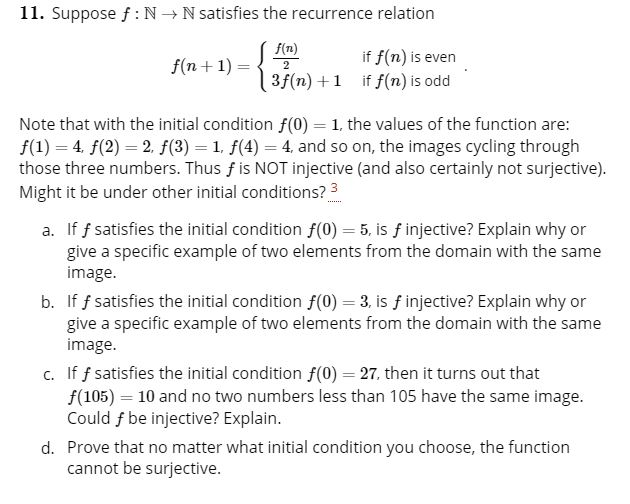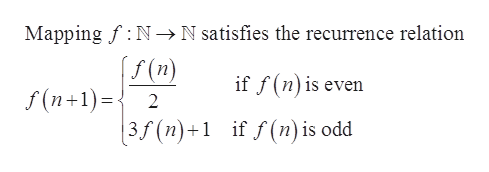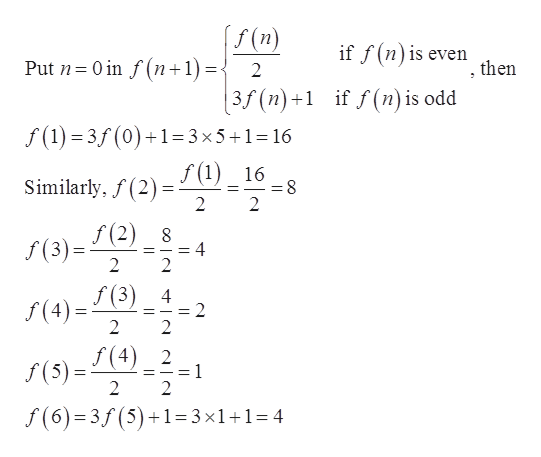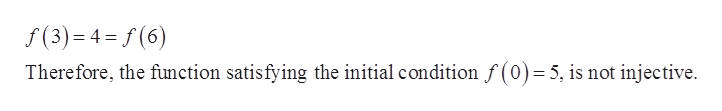# 11. Suppose f NN satisfies the recurrence relationf(n)if f(n) is even3f(n)1 if f(n) is oddf(n1)2Note that with the initial condition f(0) 1, the values of the function are:f(1) 4 f(2)2. f(3) 1. f(4) 4 and so on, the images cycling throughthose three numbers. Thus f is NOT injective (and also certainly not surjective).Might it be under other initial conditions? 3If f satisfies the initial condition f(0) 5, is finjective? Explain why orgive a specific example of two elements from the domain with the sameimage.b. If f satisfies the initial condition f(0) 3, is f injective? Explain why orgive a specific example of two elements from the domain with the sameimage.c. If f satisfies the initial condition f(0) 27, then it turns out thatf(105) 10 and no two numbers less than 105 have the same image.Could f be injective? Explain.d. Prove that no matter what initial condition you choose, the functioncannot be surjective.

Question
2 views

This question is a multi part question. A, B, and C have all been answered.  I was instructed to ask again for D to be answered.help_outlineImage Transcriptionclose11. Suppose f NN satisfies the recurrence relation f(n) if f(n) is even 3f(n)1 if f(n) is odd f(n1) 2 Note that with the initial condition f(0) 1, the values of the function are: f(1) 4 f(2)2. f(3) 1. f(4) 4 and so on, the images cycling through those three numbers. Thus f is NOT injective (and also certainly not surjective). Might it be under other initial conditions? 3 If f satisfies the initial condition f(0) 5, is finjective? Explain why or give a specific example of two elements from the domain with the same image. b. If f satisfies the initial condition f(0) 3, is f injective? Explain why or give a specific example of two elements from the domain with the same image. c. If f satisfies the initial condition f(0) 27, then it turns out that f(105) 10 and no two numbers less than 105 have the same image. Could f be injective? Explain. d. Prove that no matter what initial condition you choose, the function cannot be surjective. fullscreen
check_circle

star
star
star
star
star
1 Rating
Step 1

Given mapping and recurrence relation are,help_outlineImage TranscriptioncloseMapping f N-> N satisfies the recurrence relation f(n) if f(n) is even f(n+1) 2 if f (n) is odd | 3f (n)+1 fullscreen
Step 2

(a)If f satisfies the initial condition f (0) = 5, thenhelp_outlineImage Transcriptionclosef(n) if f(n)is even then Put n 0 in f(n 1) 2 3f (n)+1 if f(n) is odd f(1) 3f(0)1 3 x5+1=16 f (1) 16 Similarly, f(2) 2 =8 2 f(2)8 f(3) 2 2 f (3) 4 2 2 (4)(3) 2 f(4) 2 1 2 2 f(6) 3f (5)1=3x1+1 = 4 II fullscreen
Step 3

Since, the value of the function is same at di...help_outlineImage Transcriptionclosef(3) 4=f(6) Therefore, the function satisfying the initial condition f (0) 5, is not injective. fullscreen

### Want to see the full answer?

See Solution

#### Want to see this answer and more?

Solutions are written by subject experts who are available 24/7. Questions are typically answered within 1 hour.*

See Solution
*Response times may vary by subject and question.
Tagged in

### Math